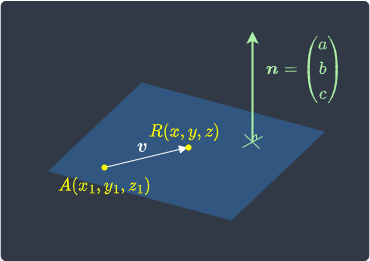search
Search
Unlock 100+ guides
search toc
close
account_circle
Profile
exit_to_app
Sign out
search
keyboard_voice
close
Searching Tips
Search for a recipe:
"Creating a table in MySQL"
Search for an API documentation: "@append"
Search for code: "!dataframe"
Apply a tag filter: "#python"
Useful Shortcuts
/ to open search panel
Esc to close search panel
to navigate between search results
d to clear all current filters
Enter to expand content previewDoc SearchCode Search BetaSORRY NOTHING FOUND!
mic
Start speaking...Voice search is only supported in Safari and Chrome.
Shrink
Navigate to

# Guide on Planes in Linear Algebra

schedule Aug 10, 2023
Last updated
local_offer
Linear Algebra
Tags
mode_heat
Master the mathematics behind data science with 100+ top-tier guides
Start your free 7-days trial now!

# Defining a plane using a point and a norm vector

Suppose we are given the following information about some plane:

• there exists a point $A$ with coordinates $(x_1,y_1,z_1)$ on the plane.

• a vector $\boldsymbol{n}$ that is perpendicular to the plane. This vector is called the norm vector.

Visually, we have the following scenario:Here, $R$ is another point on the plane with coordinates $(x,y,z)$. The difference between points $A$ and $R$ is that $A$ is assumed to be known, that is, $(x_1,y_1,z_1)$, are some fixed scalar values whereas $R$ can vary.

We know from this sectionlink that the vector pointing from $A$ to $R$, which we denote as $\boldsymbol{v}$, can be expressed as:

$$\boldsymbol{v}= \begin{pmatrix} x-x_1\\ y-y_1\\ z-z_1 \end{pmatrix}$$

From theoremlink, because $\boldsymbol{v}$ is perpendicular to the normal vector $\boldsymbol{n}$, their dot product must equal zero:

$$\begin{equation}\label{eq:NoUqoRBzM8vBpDGtLsK} \boldsymbol{n}\cdot\boldsymbol{v}=0 \end{equation}$$

Let's substitute the vectors into \eqref{eq:NoUqoRBzM8vBpDGtLsK} and simplify:

\begin{equation}\label{eq:Wy6nQjTBTpQ1iS7mdnO} \begin{aligned}[b] \begin{pmatrix} a\\b\\c \end{pmatrix}\cdot \begin{pmatrix} x-x_1\\ y-y_1\\ z-z_1 \end{pmatrix}&=0\\ a(x-x_1)+b(y-y_1)+c(z-z_1)&=0\\ ax+by+cz&=ax_1+by_1+cz_1 \end{aligned} \end{equation}

Here, the right-hand side is some constant because we assume that we are given:

• the coordinates of $A$, that is, $(x_1,y_1,z_1)$.

• the norm vector $\boldsymbol{n}$, that is, $a$, $b$ and $c$.

We now formally define two important concepts:

• the vector equation of a plane.

• the cartesian equation of a plane.

Definition.

# Vector equation of a plane

Suppose we are given that a plane:

• passes through the point $A$ represented by a position vector $\boldsymbol{a}$.

• has a normal vector $\boldsymbol{n}$.

Let $R$ be some other point on the plane represented by a position vector $\boldsymbol{r}$. The so-called vector equation of a plane is given by:

$$\begin{equation}\label{eq:YeBAPpdg7dJHKpjIrsU} \boldsymbol{n}\cdot{\boldsymbol{v}}=0 \end{equation}$$

Where $\boldsymbol{v}$ is a vector pointing from $A$ to $R$ defined as:

$$\boldsymbol{v}= \begin{pmatrix} x-x_1\\ y-y_1\\ z-z_1 \end{pmatrix} = \boldsymbol{r}- \boldsymbol{a}$$

The vector equation of a plane \eqref{eq:YeBAPpdg7dJHKpjIrsU} is also sometimes expressed as:

$$\begin{equation}\label{eq:gczzhneSgMN2ws4fZwP} \boldsymbol{n}\cdot(\boldsymbol{r}-\boldsymbol{a})=0 \end{equation}$$

In some textbooks, the vector equation is equivalently defined as:

$$\boldsymbol{n}\cdot\boldsymbol{r}= \boldsymbol{n}\cdot\boldsymbol{a}$$

Where $\boldsymbol{n}\cdot{\boldsymbol{a}}$ can be thought of as some constant.

Example.

## Finding the vector equation of a plane

Suppose a plane passes through the point $(2,4,3)$ and has the following normal vector:

$$\boldsymbol{n}= \begin{pmatrix} 5\\3\\1 \end{pmatrix}$$

Find the vector equation of a plane.

Solution. The plane is parallel to the following vector:

$$\boldsymbol{v}= \begin{pmatrix} x-2\\y-4\\z-3 \end{pmatrix}$$

The vector equation of the plane is:

$$\begin{pmatrix} 5\\3\\1 \end{pmatrix}\cdot \begin{pmatrix} x-2\\y-4\\z-3 \end{pmatrix}=0$$

Equivalently, the vector equation of the plane can be expressed as:

\begin{align*} \begin{pmatrix} 5\\3\\1 \end{pmatrix}\cdot \begin{pmatrix} x\\y\\z \end{pmatrix}&= \begin{pmatrix}5\\3\\1\end{pmatrix} \cdot \begin{pmatrix}2\\4\\3\end{pmatrix}\\ &=10+12+3\\ &=25 \end{align*}

If we let vector $\boldsymbol{r}$ be the position vector of any point $(x,y,z)$ on the plane, then the vector equation of the plane can also be compactly expressed as:

$$\boldsymbol{n}\cdot\boldsymbol{r}=25$$
Definition.

# Cartesian (general) equation of the plane

Suppose we are given that a plane passes through the point $(x_1,y_1,z_1)$ and has the normal vector $\boldsymbol{n}$ below:

$$\boldsymbol{n}= \begin{pmatrix} a\\ b\\ c \end{pmatrix}$$

The Cartesian or the general equation of the plane is:

$$ax+by+cz \;{\color{blue}=}\; ax_1+by_1+cz_1 \;{\color{blue}=}\; d$$

Where the right-hand side is to be treated as some fixed constant, say $d$.

Example.

## Finding the Cartesian equation of a plane given a point and a normal vector

Find the equation of the plane that passes through the point $(1,2,3)$ and has the normal vector below:

$$\boldsymbol{n}= \begin{pmatrix} 5\\3\\4 \end{pmatrix}$$

Solution. The cartesian equation of the plane is:

\begin{align*} 5x+3y+4z&=(5)(1)+(3)(2)+(4)(3)\\ &=23 \end{align*}
Example.

## Finding the normal vector of a plane

Consider the following equation of a plane:

$$3x+2y+4z=20$$

Determine a vector that is perpendicular to this plane.

Solution. The normal vector is the coefficients of the cartesian equation of the plane:

$$\boldsymbol{n}= \begin{pmatrix} 3\\2\\4 \end{pmatrix}$$

Note that any scalar multiple of this normal vector will also be perpendicular to the plane.

Example.

# Converting the cartesian form to the vector form

Consider the following general form of a plane:

$$2x+y+3z=5$$

Determine the vector equation of the plane.

Solution. The normal vector of the plane and the position vector of any point on the plane is:

$$\boldsymbol{n}= \begin{pmatrix} 2\\1\\3 \end{pmatrix},\;\;\;\;\; \boldsymbol{r}= \begin{pmatrix} x\\y\\z \end{pmatrix}$$

The vector equation of the plane is:

$$\boldsymbol{n} \cdot \boldsymbol{r}=5$$
thumb_up
thumb_down
Comment
Citation
Ask a question or leave a feedback...
thumb_up
0
thumb_down
0
chat_bubble_outline
0
settings
Enjoy our search
Hit / to insta-search docs and recipes!